# College Chemistry : Equilibrium

## Example Questions

2 Next →

### Example Question #2 : Le Chatelier's Principle

Consider the following equation at equilibrium: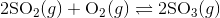What would happen if more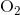were added into the equation?

There would be no change.

Less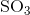would be produced.

More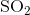would be produced.

Morewould be produced.

Morewould be produced.

Explanation:

Recall Le Chatelier's principle: A chemical system at equilibrium will shift in the direction that minimizes the disturbance to the system.

Before the addition of, the system is in equilibrium, meaning the reaction quotient is equal to the equilibrium constant;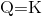.

However, after the addition of, a reactant, the reaction quotient is now less than the equilibrium constant;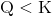.

In order to maintain equilibrium, the reaction will then shift right of favor the formation of more products.

### Example Question #3 : Le Chatelier's Principle

Consider the following exothermic equation: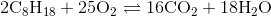Which of the following actions will cause the products to be favored?

Increase the amount of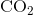.

Decrease the amount of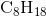.

Decrease the temperature at which the reaction is conducted.

Increase the temperature at which the reaction is conducted.

Decrease the temperature at which the reaction is conducted.

Explanation:

Since the equation is exothermic, you can think of heat as another product of the reaction: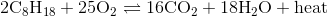Apply LeChatelier's principle to this equation.

If the temperature at which the reaction is decreased, that is akin to decreasing the amount of product made, thus causing the reaction to shift towards the products.

### Example Question #1 : Common Ion Effect

Calculate the molar solubility of barium fluoride when it is dissolved in a solution containing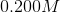of sodium fluoride. Barium fluoride has a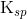value of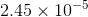.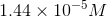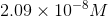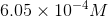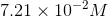Explanation:

Start by writing out the equation for the dissolution of barium fluoride.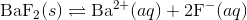Next, write out the chart to keep track of concentrations of each ion.

 [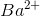] [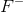] Initial 0.00 0.200 Change +x +2x Equilibrium x 0.200+2x

In the chart,is the amount of barium fluoride that dissolves. Since we are putting barium fluoride in a solution ofsodium fluoride, the initial concentration of the fluoride ion is. Since there is already some fluoride in the solution, we should expect that the molar solubility of barium fluoride should be less than its molar solubility when dissolved in water.

Now, write the equilibrium expression using the chemical equation: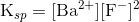Plug in the givenand solve for.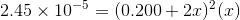At this point, use a graphing calculator to solve for.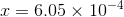The molar solubility of barium fluoride in sodium fluoride is.

### Example Question #2 : Common Ion Effect

Suppose that a chemist wants to lower the concentration of calcium in an aqueous solution by precipitating it out, according to the following reaction expression.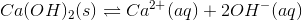Which of the following chemicals could the chemist add to the solution in order to precipitate the calcium?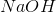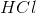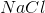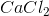Any of theseExplanation:

In this question, we're shown an equilibrium expression for a precipitation reaction in solution. We're asked to identify a compound that will help calcium precipitate out of solution.

To identify a compound that will precipitate calcium out of solution, we need to consider the equilibrium expression shown in the question stem. Applying Le Chatlier's principle, we'll need to consider the direction each of the answer choices will push the reaction in.

Addingwill make the solution more acidic. As a result, there will be a decreased amount of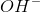. Consequently, the reaction will shift to the right and there will be more calcium in solution.

Addingwill drive up the concentration of calcium, which will drive the reaction to the left. Even though this will cause calcium to precipitate out of the solution, it nonetheless defeats the purpose because the amount of dissolved calcium in solution is not decreasing.

Addingto the solution will have no effect on the reaction equilibrium because neither of the ions appears in the expression.

Addingwill cause the solution to become more basic, with an increased amount of. Consequently, the reaction equilibrium will be driven toward the left. This will, in turn, cause calcium to precipitate out of solution in the form of the solid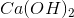.

### Example Question #1 : Solubility Product Constant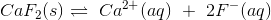Express the solubility product constant expression for the given reaction.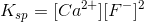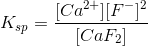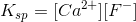Explanation:

The equilibrium given tells us how the solid dissolves in solution:However, the solubility product constant (Ksp) given tells us the degree by which a solid dissolves in solution. The larger the Ksp, the more soluble a substance is in water. Writing this expression follows the same rules as other equilibrium constant expressions. Therefore solids and water (when it is the solvent) are omitted from this expression. You must raise the concentration of the substances involved to the power of its coefficient.

For the chemical reaction given, the Ksp is:### Example Question #2 : Solubility Product Constant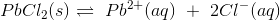Express the solubility product constant expression for the given reaction.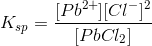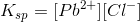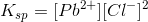Explanation:

The equilibrium given tells us how the solid dissolves in solution:However, the solubility product constant (Ksp) given tells us the degree by which a solid dissolves in solution. The larger the Ksp, the more soluble a substance is in water. Writing this expression follows the same rules as other equilibrium constant expressions. Therefore solids and water (when it is the solvent) are omitted from this expression. You must raise the concentration of the substances involved to the power of its coefficient.

For the chemical reaction given, the Ksp is:### Example Question #3 : Solubility Product Constant

How many grams of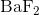are dissolved in 75.0 mL of a saturated solution of?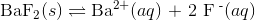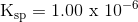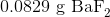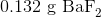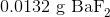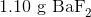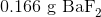Explanation:

To calculate how many grams ofare dissolved in the solution, we first solve for the molarity of the solution.

Using the dissociation equationWe can write out the equation for the solubility product constant as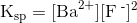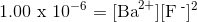Because there are 2 fluoride ions for every barium ion, we can rewrite the equation as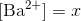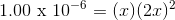Now solve for x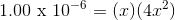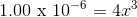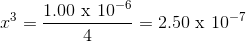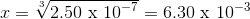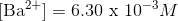Solve for the concentration of dissolved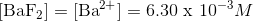Now that we have the concentration of dissolved, we can calculate how many grams are dissolved in the solution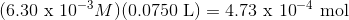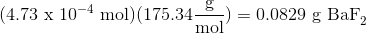2 Next →